# SI Estimate Impulse Response VI

LabVIEW 2018 Control Design and Simulation Module Help

Edition Date: March 2018

Part Number: 371894J-01

»View Product InfoDownload Help (Windows Only)

Owning Palette: Nonparametric Model Estimation VIs

Requires: Advanced Signal Processing Toolkit or Control Design and Simulation Module

Estimates the impulse response of an unknown system using the prewhitening-based correlation analysis method and the least squares method. You must manually select the polymorphic instance to use.

Use the pull-down menu to select an instance of this VI.

 Select an instance SI Estimate Impulse Response (Waveform)SI Estimate Impulse Response (Array)SI Estimate Impulse Response (Least Squares Waveform)SI Estimate Impulse Response (Least Squares Array)

## SI Estimate Impulse Response (Waveform)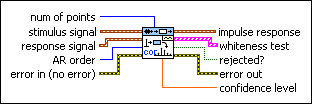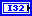num of points specifies the number of points for which to compute the impulse response.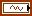stimulus signal specifies the input waveform of the stimulus signal.response signal specifies the input waveform of the response signal.AR order specifies the order of the AR model to use to estimate the filter for prewhitening. AR order must be greater than or equal to 0.error in describes error conditions that occur before this node runs. This input provides standard error in functionality.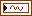impulse response returns the waveform of the estimated impulse response of the system.whiteness test displays the autocorrelation of the prewhitened stimulus signal and the 99% confidence region. If stimulus signal is well prewhitened, most of the autocorrelation, except the first point, of the whitened stimulus signal will be within the confidence region. If some points exceed the confidence region, especially the second and third points, increase AR order to improve the prewhitening.rejected? indicates whether the impulse response estimation of this VI is reliable. If rejected? is FALSE, the impulse response estimation is reliable, and the risk of accepting an incorrect impulse response estimation is 5%. If rejected? is TRUE, the impulse response estimation is not reliable. Increase AR order or redesign the experiment to improve the impulse response estimation.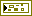error out contains error information. This output provides standard error out functionality.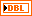confidence level returns the confidence level of the impulse response. The values of the impulse response between plus and minus confidence level are insignificant, and you can consider them to be equal to 0.

## SI Estimate Impulse Response (Array)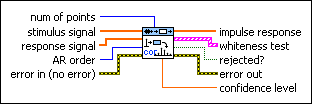num of points specifies the number of points for which to compute the impulse response.stimulus signal specifies an array that represents the stimulus signal.response signal specifies an array that represents the response signal.AR order specifies the order of the AR model to use to estimate the filter for prewhitening. AR order must be greater than or equal to 0.error in describes error conditions that occur before this node runs. This input provides standard error in functionality.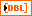impulse response returns the estimated impulse response of the unknown system.whiteness test displays the autocorrelation of the prewhitened stimulus signal and the 99% confidence region. If stimulus signal is well prewhitened, most of the autocorrelation, except the first point, of the whitened stimulus signal will be within the confidence region. If some points exceed the confidence region, especially the second and third points, increase AR order to improve the prewhitening.rejected? indicates whether the impulse response estimation of this VI is reliable. If rejected? is FALSE, the impulse response estimation is reliable, and the risk of accepting an incorrect impulse response estimation is 5%. If rejected? is TRUE, the impulse response estimation is not reliable. Increase AR order or redesign the experiment to improve the impulse response estimation.error out contains error information. This output provides standard error out functionality.confidence level returns the confidence level of the impulse response. The values of the impulse response between plus and minus confidence level are insignificant, and you can consider them to be equal to 0.

## SI Estimate Impulse Response (Least Squares Waveform)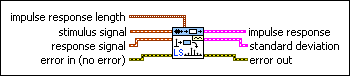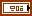impulse response length specifies the number of points for which to compute the impulse response.num of points (t<0) determines the number of data points before t=0 for which to compute the impulse response.num of points (t>=0) determines the number of data points starting from t=0 for which to compute the impulse response.stimulus signal specifies the input waveform of the stimulus signal.response signal specifies the input waveform of the response signal.error in describes error conditions that occur before this node runs. This input provides standard error in functionality.impulse response returns the estimated impulse response of the unknown system.impulse response time step returns the time step of the estimated impulse response. The time step is a time vector, in samples, against which this VI estimates the impulse response.impulse response amplitude returns the amplitude of the estimated impulse response.standard deviation returns the standard deviation of the estimated impulse response.std time step returns the time step of the standard deviation of the estimated impulse response.std amplitude returns the amplitude of the standard deviation of the estimated impulse response.error out contains error information. This output provides standard error out functionality.

## SI Estimate Impulse Response (Least Squares Array)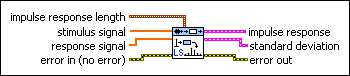impulse response length specifies the number of points for which to compute the impulse response.num of points (t<0) determines the number of data points before t=0 for which to compute the impulse response.num of points (t>=0) determines the number of data points starting from t=0 for which to compute the impulse response.stimulus signal specifies an array that represents the stimulus signal.response signal specifies an array that represents the response signal.error in describes error conditions that occur before this node runs. This input provides standard error in functionality.impulse response returns the estimated impulse response of the unknown system.impulse response time step returns the time step of the estimated impulse response. The time step is a time vector, in samples, against which this VI estimates the impulse response.impulse response amplitude returns the amplitude of the estimated impulse response.standard deviation returns the standard deviation of the estimated impulse response.std time step returns the time step of the standard deviation of the estimated impulse response.std amplitude returns the amplitude of the standard deviation of the estimated impulse response.error out contains error information. This output provides standard error out functionality.

## SI Estimate Impulse Response Details

The Correlation instances of the SI Estimate Impulse Response VI use a finite impulse response (FIR) filter to whiten the stimulus signal and compute the coefficients of the FIR filters from autoregressive (AR) processors. The AR order input determines the prewhitening behavior.

The following figure illustrates the structure of the Correlation instances of the SI Estimate Impulse Response VI. This VI conducts the prewhitening process for the stimulus signal u(k) and response signal y(k) using a pair of identical AR processors.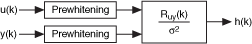In the above diagram, Ruy(k) is the cross correlation between the whitened stimulus signal u(k) and the whitened response signal y(k).is the standard deviation of the whitened stimulus signal u(k).

h(k) is the impulse response of the system.

## Examples

Refer to the following VIs for examples of using the SI Estimate Impulse Response VI:

• Estimate Impulse Response VI: labview\examples\System Identification\Getting Started\Nonparametric Estimation.llb
• Glass Tube VI: labview\examples\System Identification\Industry Applications\General.llb
• Hairdryer VI: labview\examples\System Identification\Industry Applications\Mechanical Systems.llb

Not Helpful